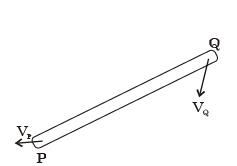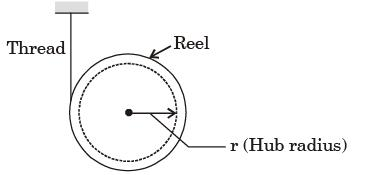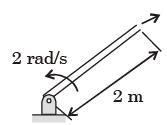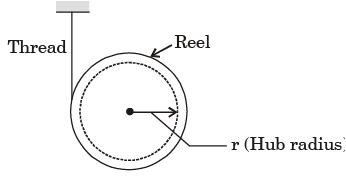Courses

# Past Year Questions: Force System Resultants Notes | EduRev

## Mechanical Engineering : Past Year Questions: Force System Resultants Notes | EduRev

The document Past Year Questions: Force System Resultants Notes | EduRev is a part of the Mechanical Engineering Course Engineering Mechanics.
All you need of Mechanical Engineering at this link: Mechanical Engineering

Question 1:A point mass is shot vertically up from ground level with a velocity of 4 m/s at time, t = 0. It loses 20% of its impact velocity after each collision with the ground. Assuming that the acceleration due to gravity is 10 m/s2 and that air resistance is negligible, the mass stops bouncing and comes to complete rest on the ground after a total time (in seconds) of



Question 2:A rigid link PQ is undergoing plane motion as shown in the figure (Vp and VQ are non-zero). VQP is the relative velocity of point Q with respect to point P.Which one of the following is TRUE?



Question 3:A circular object of radius /rolls without slipping on a horizontal level floor with the center having velocity V. The velocity at the point of contact between the object and the floor is



Question 4:A straight rod length L(t), hinged at one end freely extensible at the other end, rotates through an angle θ(t) about the hinge. At time t, L(t) = 1 m, L(t) = 1 m/s, θ(t) = p/4 rad and θ (t) = 1 rad/s.
The magnitude of the velocity at the other end of the rod is



Question 5:A reel of mass m and radius of gyration k is rolling down smoothly from rest with one end of the thread wound on it held in the ceiling as depicted in the figure.
Consider the thickness of the thread and its mass negligible in comparison with the radius r of the hub and the reel mass m. Symbol g represents the acceleration due to gravity.The tension in the thread is



Question 6:A shell is fired from a cannon. At the instant the shell is just about to leave the barrel, its velocity relative to the barrel is 3 m/s, while the barrel is swinging upwards with a constant angular velocity of 2 rad/s. The magnitude of the absolute velocity of the shell isQuestion 7:A reel of mass m and radius of gyration k is rolling down smoothly from rest with one end of the thread wound on it held in the ceiling as depicted in the figure.
Consider the thickness of the thread and its mass negligible in comparison with the radius r of the hub and the reel mass m. Symbol g represents the acceleration due to gravity.The linear acceleration of the reel is



Question 8:Instantaneous centre of a body rolling without sliding on a stationary curved surface lies.



Offer running on EduRev: Apply code STAYHOME200 to get INR 200 off on our premium plan EduRev Infinity!

## Engineering Mechanics

37 videos|85 docs|64 tests

,

,

,

,

,

,

,

,

,

,

,

,

,

,

,

,

,

,

,

,

,

;